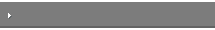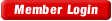HOME COURSES PREVIEW REVIEW ABOUT CONTACTToll-Free Info & Ordering M-F: 9am-5pm (PST): (877) RAPID-1024/7 Technical SupportQUICK TOURMember Login:Rapid Courses Catalog Mathematics in 24 Hours Chemistry in 24 Hours Biology in 24 Hours Physics in 24 HoursMath Survival Weekly
Get the insider's tips and tricks in how to survive your math course and ace the next test. Subscribe the Web's only math weekly newsletter for students and learn:
- How to Study Math Effectively
- How to Take Math Courses Strategically
- How to Solve Math Problems Systematically
- How to Score High in Math Exams
- How to Master Math Rapidly

Enter your name and email below and get started today!Math Study Lounge These study sheets are for quick review on the subjects. Refer to our rapid courses for comprehensive review.     - Getting Started with Algebra     - Geometry Basics     - How to Solve Math Problems     - Trigonometry Quick Review     - Statistics At-A-Glance     - Calculus PreviewHome » How to Solve Math Problems

General Problem Solving

General steps for solving any type of problem in math:

• Identify what is being given
• Clarify what is being asked
• If unclear, try to rephrase it.
• Select a strategy
• Trial & error, search, deductive reasoning, working backwards or knowledge-based
• Solve using selected strategy
• Review
• Check for reasonableness, units

Example:

• What happens to the graph of y=sin x / x as we approach x = 0 (note that it is not asking “at x=0”)?
 Step Description Information 1 Identify what’s given As x approaches “0”, what is does sin x / x approach? 2 Identify what’s being asked What does sin x / x approach? 3 Strategy: Numerical Approach Chose x values that approach to “0” – tabulate these values and corresponding sin x / x 4 Apply strategy: For x closer to x=0, evaluate f(x)5 Review The limit approaches “1” no matter how we look at it!

Clue Words:

• Clue Words for Addition: sum, total, in all, perimeter
• Clue Words for Subtraction: difference, how much more, exceed
• Clue Words for Multiplication: product, total, area, times
• Clue Words for Division: share, distribute, quotient, average

Types of Problems:

• The higher the math class, the more types of problems: in earlier classes, problems often required just one step to find a solution.
• Increasingly, you will tackle problems which require several steps to solve them.
• Break these problems down into smaller pieces and solve each piece - divide and conquer!
KUDOS = Problem Solving for Word Problems

 K nowns U nknowns D efinition O utput S ubstantiation

Known: Identify what is known

• Use units to identify information
• Write information symbolically
• Look for implied information

Unknown: Identify what is unknown

• What is the problem seeking?
• Write information symbolically

Definition: Identify the information that is needed

• Choose & rearrange equations
• Look other places for missing info
• Re-evaluate your approach if needed

Output: Find the output for your information

• Plug values into equations
• Perform the calculation

Substantiate: Check your results with common sense checks

• Check Validity
• Check Units

Example:  Find the distance traveled in 10 seconds by an object moving with a velocity of v(t) = 20 + 7 cos t meters per second.

 Step Information You Write K Time traveled t = 10 sec. Velocity function v(t) = 20 + 7 cos t U What is the distance? d = ? D Find the area under the curve v(t) = 20 + 7 cos t Graph v(t) and divide it into 5 rectangles that are 2 units wide each.  The height of each may be calculated using the function. O Add each of the 5 area’s regions 2(27.0+17.1+15.4+26.7+19.0)=210m S Check validity, units 210m is a reasonable answer and “m” is a distance unit

Math Test/Exam Tips

Preperation Checklist:

• Stay ahead of the game
• Make a cheat sheet
• Know the format & what information is “test-able”
• Make a mock-exam
• Attend the review session
• Get help early

Day of the Exam Checklist:

• Arrive early and prepared
• Listen & read instructions carefully
• Memory dump first
• Skim the test and make a plan
• Apply the guessing rule

• Scan all the choices
• Avoid the simplification trap

When Facing a Free-Response Question:

• Show partial work!
• Don’t forget the unit!
• Don’t judge length of answer on blank space
Would you like to study the entire math course in 24 hours? Master all important concepts and learn how to solve problems. Super review your upcoming exams with our cheat sheets. Get started with out rapid courses now!
Calculus
Introductory Statistics

To quickly learn about our math rapid learning system, view the movie:
 ©2014 Rapid Learning Center. Privacy Policy | Disclaimer Chemistry Survival Publishing, Biology Survival Publishing and Physics Survival Publishing are the divisions of Rapid Learning Inc.